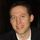cancel
Showing results for
Did you mean:Helper II

## DAX Help - Current Month * 12

Hi Guys,

I'm trying to create the following formula (YTD Leavers / FY month we are in * 12) / YTD AVR Headcount

I have created the [YTD Leavers] & [YTD AVR Headcount] measures but am not sure how to create the

[FY month we are in * 12]

I have a dedicated calendar table with month names and month numbers, our FY starts in September so its month 1

how can I have a measure that returns the month number based on the month that we are in multiplies that with 12 and returns value?

Thanks,

Kris

1 ACCEPTED SOLUTIONSuper User

Forgot the "* 12". 🙂

``````FY month we are in * 12 =
CALCULATE ( MAX ( DimDate[Month Num] ), DimDate[Date] = TODAY () ) * 12``````

Proud to be a Super User!

2 REPLIES 2Super User

Try this measure. I'm assuming that [Month Num] is the fiscal month number.

``````FY month we are in * 12 =
CALCULATE ( MAX ( DimDate[Month Num] ), DimDate[Date] = TODAY () )``````

Proud to be a Super User!Super User

Forgot the "* 12". 🙂

``````FY month we are in * 12 =
CALCULATE ( MAX ( DimDate[Month Num] ), DimDate[Date] = TODAY () ) * 12``````

Proud to be a Super User!